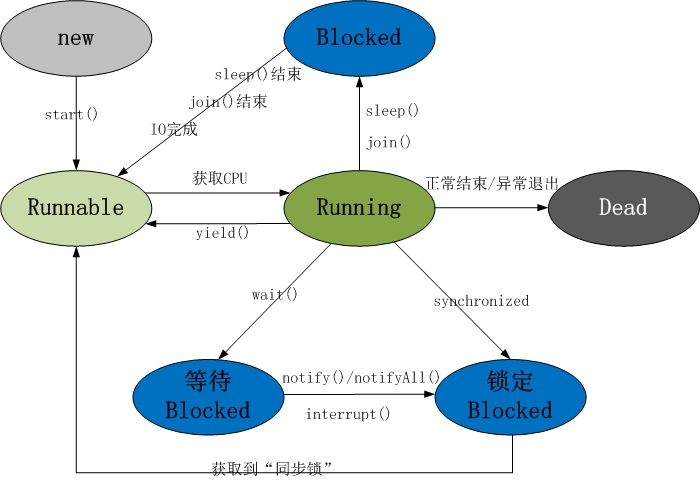# Java多线程（一）

#### 一.线程的生命周期及五种基本状态#### java线程具有五中基本状态

1.等待阻塞：运行状态中的线程执行wait()方法，使本线程进入到等待阻塞状态；

2.同步阻塞 – 线程在获取synchronized同步锁失败(因为锁被其它线程所占用)，它会进入同步阻塞状态

3.其他阻塞 – 通过调用线程的sleep()或join()或发出了I/O请求时，线程会进入到阻塞状态。当sleep()状态超时、join()等待线程终止或者超时、或者I/O处理完毕时，线程重新转入就绪状态。

#### 二. Java多线程的创建及启动

Java中线程的创建常见有如三种基本形式

 class MyThread extends Thread {

private int i = 0;

@Override
public void run() {
for (i = 0; i < 100; i++) {
}
}
}
 public class ThreadTest {

public static void main(String[] args) {
for (int i = 0; i < 100; i++) {
if (i == 30) {
}
}
}
}

class MyRunnable implements Runnable {
private int i = 0;

@Override
public void run() {
for (i = 0; i < 100; i++) {
}
}
}
 public class ThreadTest {

public static void main(String[] args) {
for (int i = 0; i < 100; i++) {
if (i == 30) {
Runnable myRunnable = new MyRunnable(); // 创建一个Runnable实现类的对象
}
}
}
}

public class ThreadTest {

public static void main(String[] args) {
for (int i = 0; i < 100; i++) {
if (i == 30) {
Runnable myRunnable = new MyRunnable();
}
}
}
}

class MyRunnable implements Runnable {
private int i = 0;

@Override
public void run() {
System.out.println("in MyRunnable run");
for (i = 0; i < 100; i++) {
}
}
}

private int i = 0;

super(runnable);
}

@Override
public void run() {
for (i = 0; i < 100; i++) {
}
}
}

Thread thread = new MyThread(myRunnable);

 public interface Runnable {
public abstract void run();
}

　@Override
public void run() {
if (target != null) {
target.run();
}
}

 public class ThreadTest {

public static void main(String[] args) {
Callable<Integer> myCallable = new MyCallable();    // 创建MyCallable对象

for (int i = 0; i < 100; i++) {
if (i == 30) {
}
}

System.out.println("主线程for循环执行完毕..");

try {
int sum = ft.get();            //取得新创建的新线程中的call()方法返回的结果
System.out.println("sum = " + sum);
} catch (InterruptedException e) {
e.printStackTrace();
} catch (ExecutionException e) {
e.printStackTrace();
}
}
}
class MyCallable implements Callable<Integer> {
private int i = 0;

// 与run()方法不同的是，call()方法具有返回值
@Override
public Integer call() {
int sum = 0;
for (; i < 100; i++) {
sum += i;
}
return sum;
}
}

public class FutureTask<V> implements RunnableFuture<V> {
//....
}     
public interface RunnableFuture<V> extends Runnable, Future<V> {

void run();
}

public class ThreadTest {

public static void main(String[] args) {

MyRunnable myRunnable = new MyRunnable();

for (int i = 0; i < 100; i++) {
if (i == 30) {
}
if(i == 40){
}
}
}
}

class MyRunnable implements Runnable {

private boolean stop;

@Override
public void run() {
for (int i = 0; i < 100 && !stop; i++) {
}
}

this.stop = true;
}
}

03-215万+07-303万+
12-31
03-1420万+
07-224255
08-163915
11-233万+